# RANDARRAY function - quick way to generate random numbers in Excel

The tutorial shows how to generate random numbers, randomly sort a list, get random selection and randomly assign data to groups. All with a new dynamic array function - RANDARRAY.

As you probably know, Microsoft Excel already has a couple of randomizing functions - RAND and RANDBETWEEN. What is the sense in introducing another one? In a nutshell, because it's far more powerful and can replace both older functions. Apart from setting up your own maximum and minimum values, it lets you specify how many rows and columns to fill and whether to produce random decimals or integers. Used together with other functions, RANDARRAY can even shuffle data and pick a random sample.

## Excel RANDARRAY function

The RANDARRAY function in Excel returns an array of random numbers between any two numbers that you specify.

It is one of six new dynamic array functions introduced in Microsoft Excel 365. The result is a dynamic array that spills into the specified number of rows and columns automatically.

The function has the following syntax. Please notice that all the arguments are optional:

RANDARRAY([rows], [columns], [min], [max], [whole_number])

Where:

Rows (optional) - defines how many rows to fill. If omitted, defaults to 1 row.

Columns (optional) - defines how many columns to fill. If omitted, defaults to 1 column.

Min (optional) - the smallest random number to produce. If not specified, the default 0 value is used.

Max (optional) - the largest random number to create. If not specified, the default 1 value is used.

Whole_number (optional) - determines what kind of values to return:

• TRUE - whole numbers
• FALSE or omitted (default) - decimal numbers

### RANDARRAY function - things to remember

To efficiently generate random numbers in your Excel worksheets, there are 6 important points to take notice of:

• The RANDARRAY function is only available in Excel for Microsoft 365 and Excel 2021. In Excel 2019, Excel 2016 and earlier versions the RANDARRAY function is not available.
• If the array returned by RANDARRAY is the final result (output in a cell and not passed to another function), Excel automatically creates a dynamic spill range and populates it with the random numbers. So, be sure you have enough empty cells down and/or to the right of the cell where you enter the formula, otherwise a #SPILL error will occur.
• If none of the arguments is specified, a RANDARRAY() formula returns a single decimal number between 0 and 1.
• If the rows or/and columns arguments are represented by decimal numbers, they will be truncated to the whole integer before the decimal point (e.g. 5.9 will be treated as 5).
• If the min or max argument is not defined, RANDARRAY defaults to 0 and 1, respectively.
• Like other random functions, Excel RANDARRAY is volatile, meaning it generates a new list of random values every time the worksheet is calculated. To prevent this from happening, you can replace formulas with values by using Excel's Paste Special > Values feature.

## Basic Excel RANDARRAY formula

And now, let me show you a random Excel formula in its simplest form.

Supposing you want to fill a range consisting of 5 rows and 3 columns with any random numbers. To have it done, set up the first two arguments this way:

• Rows is 5 since we want the results in 5 rows.
• Columns is 3 as we want the results in 3 columns.

All of the other arguments we leave to their default values and get the following formula:

`=RANDARRAY(5, 3)`

Enter it in the top left cell of the destination range (A2 in our case), press the Enter key, and you will have the results spilt over the specified number of rows and columns.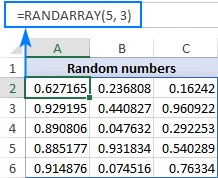As you can see in the screenshot above, this basic RANDARRAY formula fills the range with random decimal numbers from 0 to 1. If you'd rather get whole numbers within a specific range, then configure the last three arguments as demonstrated in further examples.

## How to randomize in Excel - RANDARRAY formula examples

Below you will find a few advanced formulas that cover typical randomizing scenarios in Excel.

### Generate random numbers between two numbers

To create a list of random numbers within a specific range, supply the minimum value in the 3rd argument and the maximum number in the 4th argument. Depending on whether you need integers or decimals, set the 5th argument to TRUE or FALSE, respectively.

As an example, let's populate a range of 6 rows and 4 columns with random integers from 1 to 100. For this, we set up the following arguments of the RANDARRAY function:

• Rows is 6 since we want the results in 6 rows.
• Columns is 4 as we want the results in 4 columns.
• Min is 1, which is the minimum value we wish to have.
• Max is 100, which is the maximum value to be generated.
• Whole_number is TRUE because we need integers.

Putting the arguments together, we get this formula:

`=RANDARRAY(6, 4, 1, 100, TRUE)`

And it produces the following result: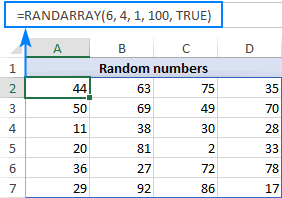### Generate random date between two dates

Looking for a random date generator in Excel? The RANDARRAY function is an easy solution! All you have to do is input the earlier date (date 1) and later date (date 2) in predefined cells, and then reference those cells in your formula:

RANDARRAY(rows, columns, date1, date2, TRUE)

For this example, we have created a list of random dates between the dates in D1 and D2 with this formula:

`=RANDARRAY(10, 1, D1, D2, TRUE)`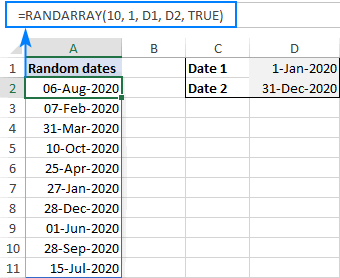Of course, nothing prevents you from supplying the min and max dates directly in the formula if you wish to. Just be sure you enter them in the format that Excel can understand:

`=RANDARRAY(10, 1, "1/1/2020", "12/31/2020", TRUE)`

To prevent mistakes, you can use the DATE function for entering dates:

`=RANDARRAY(10, 1, DATE(2020,1,1), DATE(2020,12,31), TRUE)`

Note. Internally Excel stores dates as serial numbers, so the formula results will most likely be displayed as numbers. To display the results correctly, apply the Date format to all the cells in the spill range.

### Generate random workdays in Excel

To produce random working days, embed the RANDARRAY function in the first argument of WORKDAY like this:

WORKDAY(RANDARRAY(rows, columns, date1, date2, TRUE), 1)

RANDARRAY will create an array of random start dates, to which the WORKDAY function will add 1 workday and ensure that all the returned dates are working days.

With date 1 in D1 and date 2 in D2, here's the formula to produce a list of 10 weekdays:

`=WORKDAY(RANDARRAY(10, 1, D1, D2, TRUE), 1)`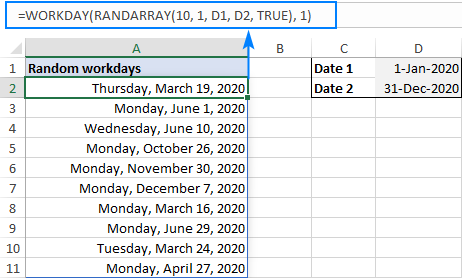As with the previous example, please remember to format the spill range as Date to have the results displayed correctly.

### How to generate random numbers without duplicates

Though modern Excel offers 6 new dynamic array functions, unfortunately, there is still no inbuilt function to return random numbers without duplicates.

To build your own unique random number generator in Excel, you will need to chain several functions together like shown below.

Random integers:

INDEX(UNIQUE(RANDARRAY(n*2, 1, min, max, TRUE)), SEQUENCE(n))

Random decimals:

INDEX(UNIQUE(RANDARRAY(n*2, 1, min, max, FALSE)), SEQUENCE(n))

Where:

• N is how many values you wish to generate.
• Min is the lowest value.
• Max is the highest value.

For example, to produce 10 random whole numbers with no duplicates, use this formula:

`=INDEX(UNIQUE(RANDARRAY(20, 1, 1, 100, TRUE)), SEQUENCE(10))`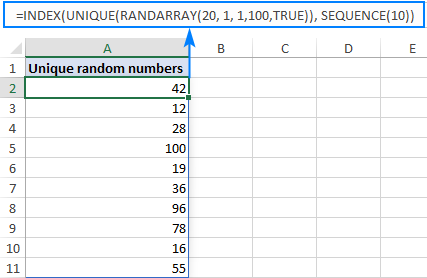To create a list of 10 unique random decimal numbers, change TRUE to FALSE in the last argument of the RANDARRAY function or simply omit this argument:

`=INDEX(UNIQUE(RANDARRAY(20, 1, 1, 100, FALSE)), SEQUENCE(10))`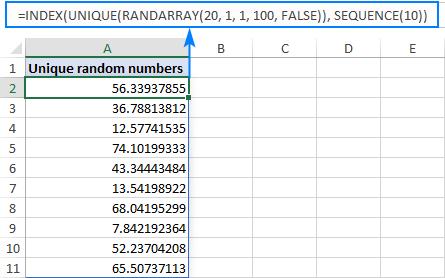Tips and notes:

### How to randomly sort in Excel

To shuffle data in Excel, use RANDARRAY for the "sort by" array (by_array argument) of the SORTBY function. The ROWS function will count the number of rows in your data set, indicating how many random numbers to generate:

SORTBY(data, RANDARRAY(ROWS(data)))

With this approach, you can randomly sort a list in Excel, whether it contains numbers, dates or text entries:

`=SORTBY(A2:A13, RANDARRAY(ROWS(A2:A13)))`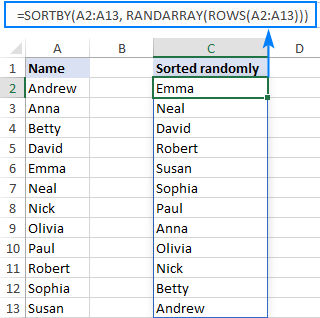Also, you can also shuffle rows without mixing your data:

`=SORTBY(A2:B10, RANDARRAY(ROWS(A2:B10)))`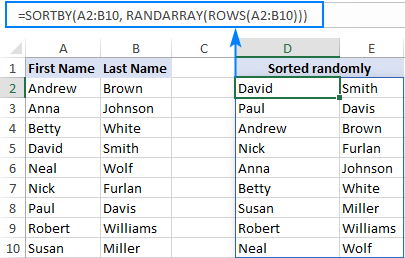### How to get a random selection in Excel

To extract a random sample from a list, here's a generic formula to use:

INDEX(data, RANDARRAY(n, 1, 1, ROWS(data), TRUE))

Where n is the number of random entries you wish to extract.

For example, to randomly select 3 names from the list in A2:A10, use this formula:

`=INDEX(A2:A10, RANDARRAY(3, 1, 1, ROWS(A2:A10), TRUE))`

Or input the desired sample size in some cell, say C2, and reference that cell:

`=INDEX(A2:A10, RANDARRAY(C2, 1, 1, ROWS(A2:A10), TRUE))`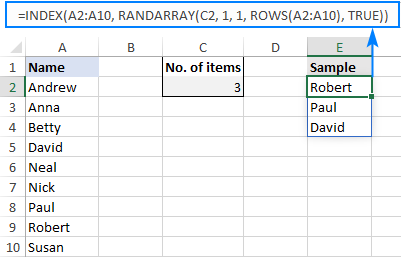How this formula works:

At the core of this formula is the RANDARRAY function that creates a random array of integers, with the value in C2 defining how many values to generate. The minimal number is hardcoded (1) and the maximum number corresponds to the number of rows in your data set, which is returned by the ROWS function.

The array of random integers goes directly to the row_num argument of the INDEX function, specifying the positions of the items to return. For the sample in the screenshot above, it is:

`=INDEX(A2:A10, {8;7;4})`

Tip. When picking a big sample from a small data set, chances are that your random selection will contain more than one occurrence of the same entry, because there is no guarantee that RANDARRAY will produce only unique numbers. To prevent this from happening, use a duplicate-free version of this formula.

### How to select random rows in Excel

If your data set contains more than one column, then specify which columns to include in the sample. For this, supply an array constant for the last argument (column_num) of the INDEX function, like this:

`=INDEX(A2:B10, RANDARRAY(D2, 1, 1, ROWS(A2:A10), TRUE), {1,2})`

Where A2:B10 is the source data and D2 is the sample size.

As the result, our random selection will contain two columns of data: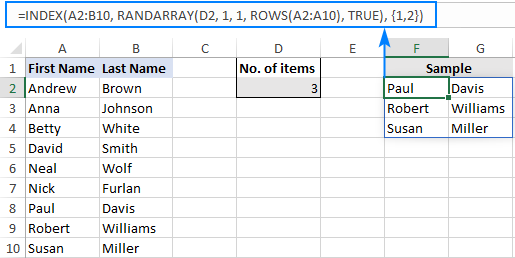Tip. As is the case with the previous example, this formula may return duplicate records. To ensure that your sample has no repeats, use a slightly different approach described in How to select random rows without duplicates.

### How to randomly assign numbers and text in Excel

To do random assignment in Excel, use RANDBETWEEN together with the CHOOSE function in this way:

CHOOSE(RANDARRAY(ROWS(data), 1, 1, n, TRUE), value1, value2,…)

Where:

• Data is a range of your source data to which you want to assign random values.
• N is the total number of values to assign.
• Value1, value2, value3, etc. are the values to be assigned randomly.

For example, to assign numbers from 1 to 3 to participants in A2:A13, use this formula:

`=CHOOSE(RANDARRAY(ROWS(A2:A13), 1, 1, 3, TRUE), 1, 2, 3)`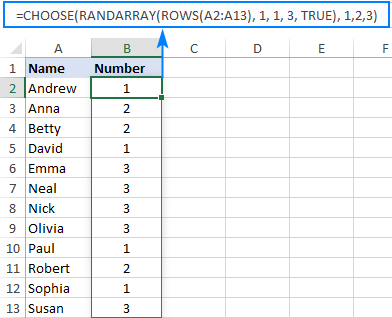For convenience, you can enter the values to assign in separate cells, say from D2 to D4, and reference those cells in your formula (individually, not as a range):

`=CHOOSE(RANDARRAY(ROWS(A2:A13), 1, 1, 3, TRUE), D2, D3, D4)`

As the result, you will be able to randomly assign any numbers, letters, text, dates and times with the same formula: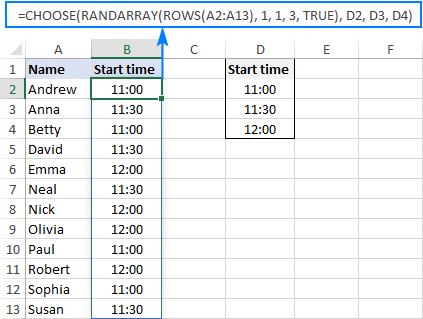Note. The RANDARRAY function will keep generating new random values with every change in the worksheet, as the result new values will be assigned every time. To "fix" the assigned values, use the Paste Special > Values features to replace formulas with their calculated values.

How this formula works

At the heart of this solution is again the RANDARRAY function that produces an array of random integers based on the min and max numbers that you specify (from 1 to 3 in our case). The ROWS function tells RANDARRAY how many random numbers to generate. This array goes to the index_num argument of the CHOOSE function. For example:

`=CHOOSE({1;2;1;2;3;2;3;3;1;3;1;2}, D2, D3, D4)`

Index_num is the argument that determines the positions of the values to return. And because the positions are random, the values in D2:D4 are picked in a random order. Yep, it's that simple :)

### How to randomly assign data to groups

When your task is to randomly assign participants to groups, the above formula may not be suitable because it does not control how many times a given group is chosen. For example, 5 persons could be assigned to group A while only 2 persons to group C. To do random assignment evenly, so that each group has the same number of participants, you need a different solution.

First, you generate a list of random numbers by using this formula:

`=RANDARRAY(ROWS(A2:A13))`

Where A2:A13 are your source data.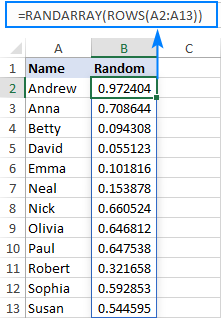And then, you assign groups (or anything else) by using this generic formula:

INDEX(values_to_assign, ROUNDUP(RANK(first_random_number, random_numbers_range)/n, 0))

Where n is the group size, i.e. the number of times each value should be assigned.

For example, to randomly assign people to the groups listed in E2:E5, so that each group has 3 participants, use this formula:

`=INDEX(\$E\$2:\$E\$5, ROUNDUP(RANK(B2,\$B\$2:\$B\$13)/3,0))`

Please notice that it's a regular formula (not a dynamic array formula!), so you need to lock the ranges with absolute references like in the above formula.

Enter your formula in the top cell (C2 in our case) and then drag it down to as many cells as needed. The result will look similar to this: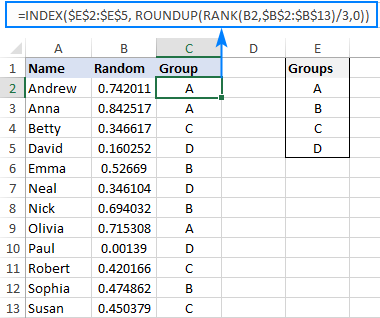Please remember that the RANDARRAY function is volatile. To prevent generating new random values every time you change something in the worksheet, replace formulas with their values by using the Paste Special feature.

How this formula works:

The RANDARRAY formula in the helper column is very simple and hardly requires explanation, so let us focus on the formula in column C.

`=INDEX(\$E\$2:\$E\$5, ROUNDUP(RANK(B2,\$B\$2:\$B\$13)/3,0))`

The RANK function ranks the value in B2 against the array of random numbers in B2:B13. The result is a number between 1 and the total number of participants (12 in our case).

The rank is divided by the group size, (3 in our example), and the ROUNDUP function rounds it up to the nearest integer. The result of this operation is a number between 1 and the total number of groups (4 in this example).

The integer goes to the row_num argument of the INDEX function, forcing it to return a value from the corresponding row in the range E2:E5, which represents the assigned group.

## Excel RANDARRAY function not working

When your RANDARRAY formula returns an error, these are the most obvious reasons to check:

### #SPILL error

As with any other dynamic array function, a #SPILL! error most often means that there isn't enough space in the intended spill range to display all the results. Just clear all the cells in this range, and your formula will recalculate automatically. For more information, please see Excel #SPILL error - causes and fixes.

### #VALUE error

A #VALUE! error may occur in these circumstances:

• If a max value is less than a min value.
• If any of the arguments is non-numeric.

### #NAME error

In most cases, a #NAME! error indicates one of the following:

• The function's name is misspelled.
• The function is not available in your Excel version.

### #CALC! error

A #CALC! error occurs if the rows or columns argument is less than 1 or refers to a blank cell.

That's how to build a random number generator in Excel with the new RANDARRAY function. I thank you for reading and hope to see you on our blog next week!War in Ukraine. Here's what Ablebits is doing to make sure our team and projects are safe.